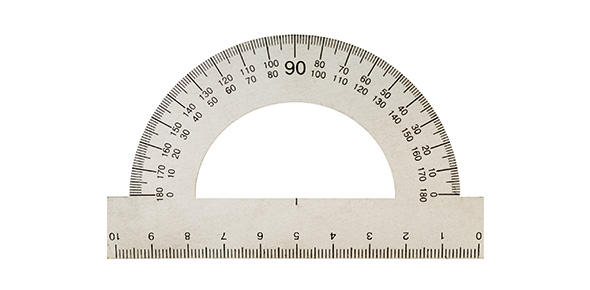# Measurement In Science

5 Questions | Total Attempts: 1693SettingsThis is a quiz for middle school to test their knowledge on scientific measurement.

Related Topics
• 1.
We use a meter stick to measure _____________.
• A.

Volume

• B.

Temperature

• C.

Time

• D.

Specific heat

• E.

Length

• 2.
In science class we use _____________ units.
• A.

SI

• B.

Imperial

• 3.
Liters are used to measure ____________.
• A.

Volume

• B.

Length

• C.

Time

• D.

Mass

• 4.
We use a balance to measure mass. What is the SI unit for mass?
• A.

Kiloliter

• B.

Nanometer

• C.

Gram

• D.

Milli

• 5.
When measuring temperature in SI units we use __________.
• A.

Celsius

• B.

Fahrenheit